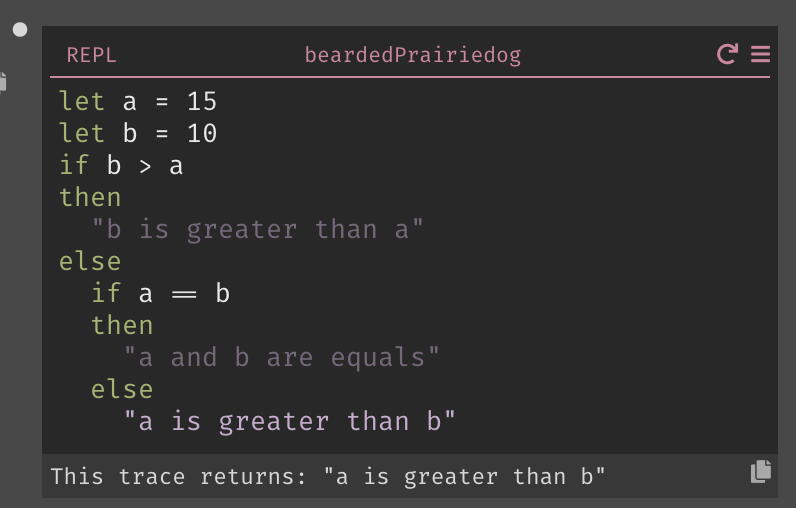# Python Cheat Sheet

This guide will help you learn Darklang if you're familiar with Python.

Have a tip to add to the guide? Submit a PR!

In Python, you use a `#` to start a comment:

``# This is a comment``

In Darklang, you use a `let _ =` to start a comment, and you write the comment as a String: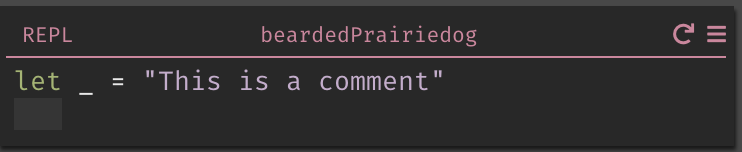## Strings​

In Python, you can write a String in multiple ways:

``"This is a string"'This is also a string'``

In Darklang, you always use double quotes: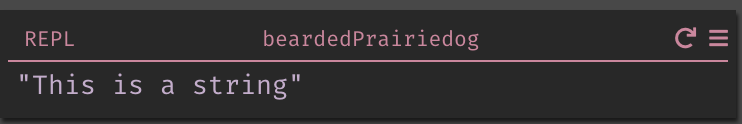## Functions​

In Python, you use the `def` keyword to define a function:

``def say_hello():    print('Hello World')``

and you call a function with the function name, followed by parentheses:

``say_hello()``

In Darklang, you create a function from the sidebar: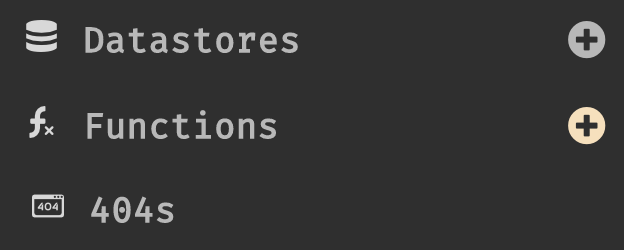This will open a new function in the function space, where you can define the name of the function and its contents: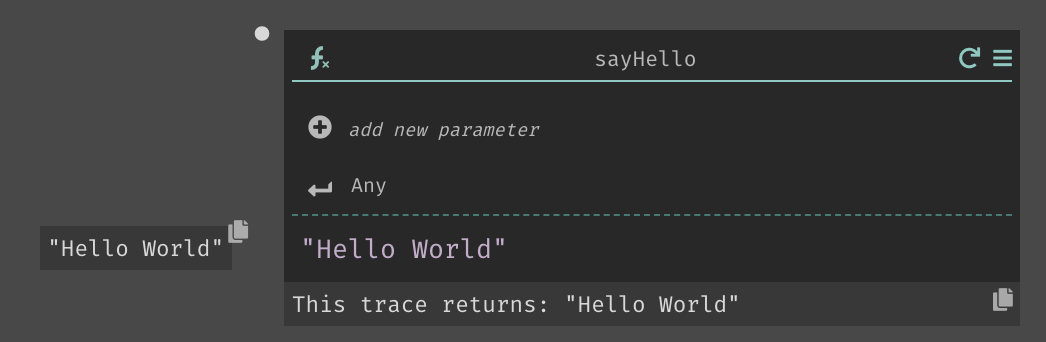## Standard Library/Built-in Functions​

Python defines a set of built in functions that are called like this:

``# This returns 2 to the power of 2.math.power(2,2)``

Darklang has a set of built in functions as well, which can be accessed via the autocomplete: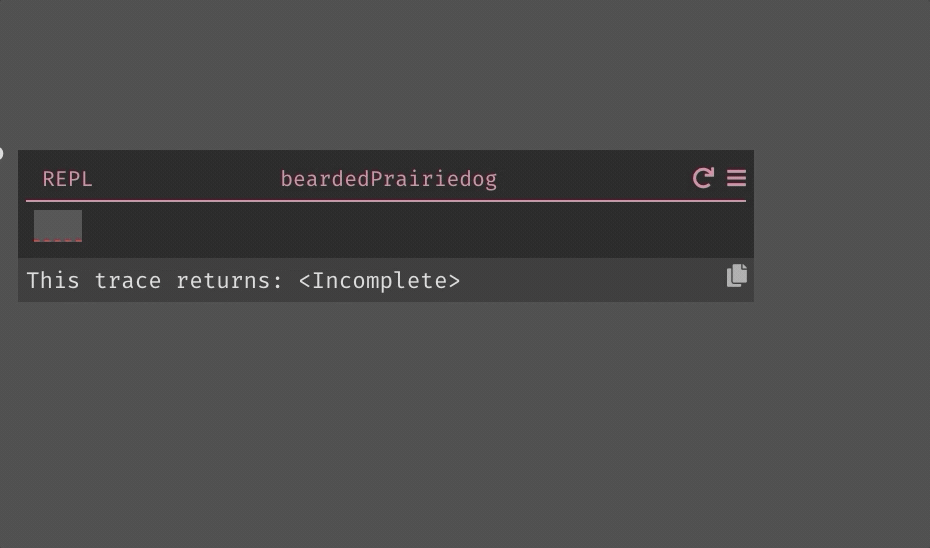## If/Elif/Else statements​

In Python, you would write an `if` statement like this:

``a = 5b = 10if p > a:   print("b is greater than a")elif a == b:   print("a and b are equal")else    print("a is greater than b")``

In Darklang, the same statement would look this this: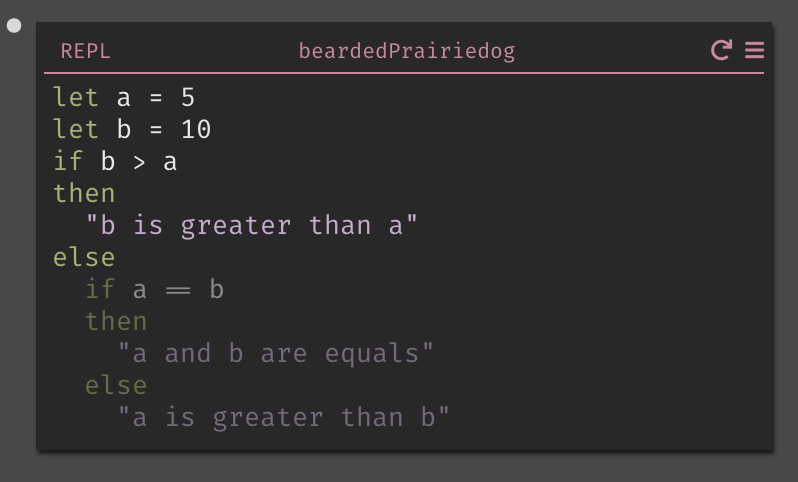Note that we highlight the path your code takes - so if we were to change the values, a different piece of the `if` statement would be highlighted: